## 元气骑士武器怎么合成-元气骑士合成表大全最近有很多萌新玩家表示还不太清楚元气骑士武器怎么合成?那么接下来了小编就为的玩家们带来了元气骑士武器合成表大全，希望可以帮助到各位玩家哦。

强力分裂者+绿色响尾蛇=蝰蛇(攻击后子弹爆开附带跟踪效果带暴击毒伤)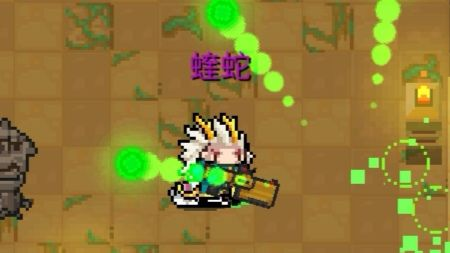光剑红+光剑蓝=光剑紫(可以普攻可以蓄力，蓄力后进行扇形平移扫射)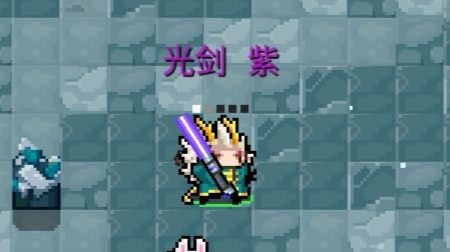火箭筒(不是火箭炮)+冰川=冰爆(射出后炸裂出一块冰屑，并在地上残留一段时间)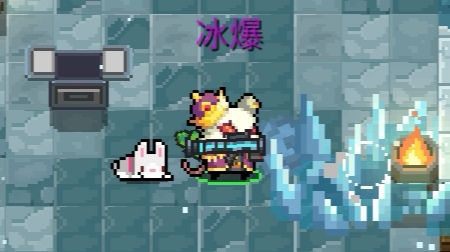大雪狐+弩=灰狐(双联发弩)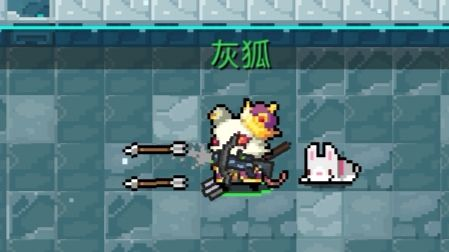外星之鹰+粒子之鹰=等离子之鹰(弹射类大光球暴击附带闪电标记，再攻击附带伤害)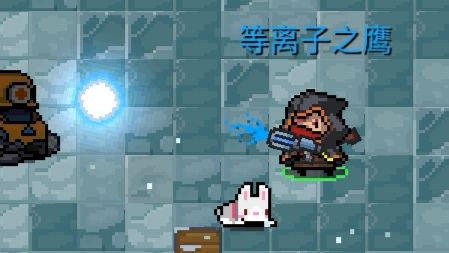1、雪狐系列

雪狐+雪狐=大雪狐

大雪狐+大雪狐=巨雪狐

巨雪狐+巨雪狐=巨巨雪狐

雪狐土豪金+樱花霰弹枪=雪狐玫瑰金

2、时代系列

欠欠时代冲锋枪+欠欠时代冲锋枪=欠时代时代冲锋枪

欠时代冲锋枪+欠时代冲锋枪=次时代冲锋枪

次时代冲锋枪+次时代冲锋枪=次次时代冲锋枪

二次时代冲锋枪+二次时代冲锋枪=三次时代冲锋枪

三次时代冲锋枪+三次时代冲锋枪=四次时代冲锋枪

3、黄金喷漆

狙击步枪+黄金喷漆=黄金狙击步枪

左轮+黄金喷漆=黄金左轮

精致法杖+黄金喷漆=黄金精致法杖

法杖+黄金喷漆=黄金法杖

沙漠之鹰+黄金喷漆=黄金沙漠之鹰

阿卡47+黄金喷漆=黄金阿卡47

4、蓝色喷漆

加特林+蓝色喷漆=蓝火加特林

织网者=蓝色喷漆=剧毒织网者

5、进阶套件

法杖+进阶套件=精致法杖

雪狐+进阶套件=大雪狐

大雪狐+进阶套件=巨雪狐

巨雪狐+进阶套件=巨巨雪狐

巨雪狐+进阶套件=雪狐土豪金

雪狐土豪金+进阶套件=雪狐玫瑰金

欠欠时代冲锋枪+进阶套件=欠时代冲锋枪

欠时代冲锋枪+进阶套件=次时代冲锋枪

次时代冲锋枪+进阶套件=次次时代冲锋枪

次次时代冲锋枪+进阶套件=次次次时代冲锋枪

次次次时代冲锋枪+进阶套件=次次次时代冲锋枪

冲锋枪一代+进阶套件冲锋枪二代

冲锋枪二代+进阶套件=冲锋枪三代

冲锋枪三代+进阶套件=冲锋枪四代

霞弹枪一代+进阶套件=霞弹枪二代

霞弹枪二代+进阶套件=霞弹枪三代

初始武器+进阶套件=初始武器进阶版

离子轨道炮+进阶套件=离子轨道炮二代

守卫者轨道炮+进阶套件=电磁轨道炮

黄金狙击步枪+进阶套件=黄金狙击枪专业版

雷电战锤+进阶套件=雷暴战锤

武士刀+进阶套件=40米长刀

短截散弹枪+进阶套件=超级散弹枪

光之杖+进阶套件=女神锡杖

攻城弩+进阶套件=床子弩

6、便携式烤炉

大葱+便携式烤炉=烤大葱

萝卜+便携式烤炉=烤萝卜

肉+便携式烤炉=烤肉

鱼+便携式烤炉=烤鱼

尚方宝剑+便携式烤炉=烤尚方宝剑

激光鱼+便携式烤炉=烤激光鱼

7、时间机器

双刃剑+时间机器=光剑红

武士刀+时间机器=光剑蓝

改良冲锋枪+时间机器=次时代冲锋枪

破旧的手枪+时间机崭新的手枪

老旧的狙击枪+时间机器=狙击步枪

老旧的火箭筒+时间机器=火箭筒

老旧的卡宾枪+时间机器=美国卡宾枪

大巫师的旧法杖+时间机器=大巫师之杖

8、种田

萝卜成熟后再生长一次=百年萝卜

竹子成熟后再生长一次=千年笋

9、手枪

两把不同應(雪人不算)+樱花霞弹枪=喂嘤鷹

冰霜之鹰+火焰之鹰=冰与火之鹰

p250+p250=双管手枪

破旧的手枪4=妙妙枪

10、步枪

杀猪刀+狙击步枪=刺枪

杀猪刀+老旧的狙击枪=刺枪

杀猪刀+黄金狙击步枪=刺枪

杀猪刀+黄金狙击枪专业版=刺枪

熔炉+冰川=极光

冲锋枪一代+冲锋枪一代=螺旋冲锋枪

冲锋枪三代+冲锋枪三代=冲锋枪四代

突击步枪+突击步枪=强化突击步枪

强化突击步枪+强化突击步枪=强化突击步枪

强化突击步枪+仲裁者=突击步枪战术精英版(雷电)

强化突击步枪+审判者=突击步枪战术精英版(眩晕)

强化突击步枪+碎冰者=突击步枪战术精英版(冰冻)

强化突击步枪+可恶激光枪=突击步枪战术精英版(剧毒)

强化突击步枪+火箭炮=榴弹机枪

11、霞弹

红黄蓝冲锋枪+骑士之拳=彩虹

骑士之拳+闪电法杖=天堂之拳

彩虹+加特林=彩虹加特林

红色响尾蛇绿色响尾蛇=沙罗曼蛇

12、导弹

喇叭+火箭炮=聚爆

13、激光

网瘾少年+网瘾少女=羊叫兽

审判者+审判者=散射激光枪

14、弓望

英雄弓+风之弓=魔法弓

火焰弓+瑶之弓+冰霜弓=群星之弓

弓/强弓+弓/强弓=巨弓

督+弩=攻城弩

攻城弩+TNT=爆炸弩

弩+TNT=爆炸弩

15、法杖

大葱+木棍=自然法杖

大葱+浪潮法杖=有机后浪

16、近战

战斧+火之杖=火焰战斧

木棍+斧子=长柄斧

火焰剑+冰霜剑=雷剑

光剑红光剑蓝+光剑绿=光剑土豪金

破碎的剑柄+破碎的剑刀=重铸的圣剑

浪潮法杖+干草叉=三叉戟

仲裁者+标枪=激光枪

锤子+TNT=爆炸战锤

17、投掷

冰锥+标枪=冰枪

手里剑+手里剑=风魔手里剑

风魔手里剑+风魔手里剑=风之手里剑

电弧手里剑+电弧手里剑=量子手里剑

雷电战锤+电弧手里剑=宙斯之矛

千本+外星之鹰=千本喂(眩晕)

千本+剧毒之鹰=千本喂(剧毒)

千本+火焰之鹰=干本喂(灼烧)

千本+冰霜之鹰=千本喂(冰冻)

干本+粒子之鹰=干本喂(雷电)

18、生活

塞子+塞子=拔粪宝

无尽能量药水+无尽生命药水=无尽恢复药水

鱼+碎冰者=激光鱼

四种特殊合成机制介绍

第一种

武器+喷漆配件=新武器

第二种

食品武器+便携式烤炉配件=熟了的可食用武器

第四种

种在花圃内的成熟植物+反复浇水=进阶植物

以上就是小编带来的元气骑士武器合成表大全，感兴趣的朋友不要错过哦。